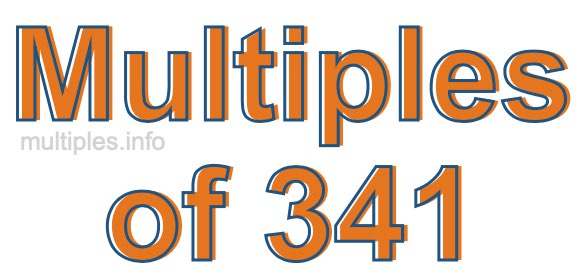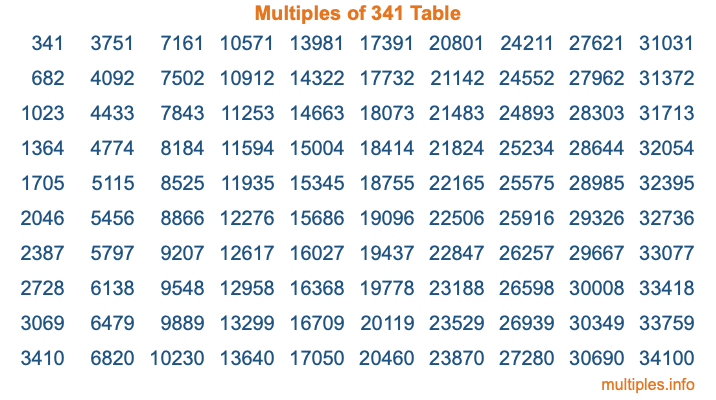Multiples of 341Welcome to the Multiples of 341 page. Here we will first teach you everything you will ever need to know about the multiples of 341, and then give you a study guide summary of everything we taught you to make sure you remember it all. Use this page to look up facts and learn information about the multiples of 341. This page will make you a multiples of three hundred forty-one expert!

Definition of Multiples of 341
Multiples of 341 are all the numbers that when divided by 341 equal an integer. Each of the multiples of 341 are called a multiple. A multiple of 341 is created by multiplying 341 by an integer.

Therefore, to create a list of multiples of 341, you start with 1 multiplied by 341, then 2 multiplied by 341, then 3 multiplied by 341, and so on for as long as you want. Thus, the list of the first five multiples of 341 is 341, 682, 1023, 1364, and 1705. To see a larger list of multiples of 341, see the printable image of Multiples of 341 further down on this page. We also have a category where you can choose any nth multiple of 341.

Multiples of 341 Checker
The Multiples of 341 Checker below checks to see if any number of your choice is a multiple of 341. In other words, it checks to see if there is any number (integer) that when multiplied by 341 will equal your number. To do that, we divide your number by 341. If the the quotient is an integer, then your number is a multiple of 341.

Is  a multiple of 341?

Least Common Multiple of 341 and ...
A Least Common Multiple (LCM) is the lowest multiple that two or more numbers have in common. This is also called the smallest common multiple or lowest common multiple and is useful to know when you are adding our subtracting fractions. Enter one or more numbers below (341 is already entered) to find the LCM.

Check out our LCM Calculator if you need more details about the Least Common Multiple or if you need the LCM for different numbers for adding and subtraction fractions.

nth Multiple of 341
As we stated above, 341 is the first multiple of 341, 682 is the second multiple of 341, 1023 is the third multiple of 341, and so on. Enter a number below to find the nth multiple of 341.

th multiple of 341

Multiples of 341 vs Factors of 341
341 is a multiple of 341 and a factor of 341, but that is where the similarities end. All postive multiples of 341 are 341 or greater than 341. All positive factors of 341 are 341 or less than 341.

Below is the beginning list of multiples of 341 and the factors of 341 so you can compare:

Multiples of 341: 341, 682, 1023, 1364, 1705, etc.

Factors of 341: 1, 11, 31, 341

As you can see, the multiples of 341 are all the numbers that you can divide by 341 to get a whole number. The factors of 341, on the other hand, are all the whole numbers that you can multiply by another whole number to get 341.

It's also interesting to note that if a number (x) is a factor of 341, then 341 will also be a multiple of that number (x).

Multiples of 341 vs Divisors of 341
The divisors of 341 are all the integers that 341 can be divided by evenly. Below is a list of the divisors of 341.

Divisors of 341: 1, 11, 31, 341

The interesting thing to note here is that if you take any multiple of 341 and divide it by a divisor of 341, you will see that the quotient is an integer.

Multiples of 341 Table
Below is an image of the first 100 multiples of 341 in a table. The table is in chronological order, column by column. The first column has the first ten multiples of 341, the second column has the next ten multiples of 341, and so on.The Multiples of 341 Table is also referred to as the 341 Times Table or Times Table of 341. You are welcome to print out our table for your studies.

Negative Multiples of 341
Although not often discussed or needed in math, it is worth mentioning that you can make a list of negative multiples of 341 by multiplying 341 by -1, then by -2, then by -3, and so on, to get the following list of negative multiples of 341:

-341, -682, -1023, -1364, -1705, etc.

Multiples of 341 Summary
Below is a summary of important Multiples of 341 facts that we have discussed on this page. To retain the knowledge on this page, we recommend that you read through the summary and explain to yourself or a study partner why they hold true.

There are an infinite number of multiples of 341.

A multiple of 341 divided by 341 will equal a whole number.

341 divided by a factor of 341 equals a divisor of 341.

The nth multiple of 341 is n times 341.

The largest factor of 341 is equal to the first positive multiple of 341.

341 is a multiple of every factor of 341.

341 is a multiple of 341.

A multiple of 341 divided by a divisor of 341 equals an integer.

341 divided by a divisor of 341 equals a factor of 341.

Any integer times 341 will equal a multiple of 341.

Multiples of a Number
Here you can get the multiples of another number, all with the same attention to detail as we did for multiples of 341 on this page.

Multiples of
Multiples of 342
Did you find our page about multiples of three hundred forty-one educational? Do you want more knowledge? Check out the multiples of the next number on our list!

Copyright  |   Privacy Policy  |   Disclaimer  |   Contact